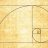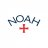# VWAP label with upper/lower bands#### J007RMC

##### Well-known member
2019 Donor
VIP
Code:
``````#HINT: The Volume-Weighted Average Price (VWAP) is calculated where size x is the volume traded at price x.
# The VWAP plot is accompanied with two bands serving as overbought and oversold levels. The Upper band (overbought level) is plotted a specified number of standard deviations above the VWAP, and the Lower band (oversold level) is plotted similarly below the VWAP. Standard deviations are based upon the difference between the price and VWAP.
input numDevDn = -2.0;
input numDevUp = 2.0;
input timeFrame = {default DAY, WEEK, MONTH};
input show_VWAP_label = yes;
input VWAP_Label_Color_Choice = {default "magenta", "green", "pink", "cyan", "orange", "red", "blue", "gray", "violet"};
input show_VWAP_bands_label = no;
input Bands_Label_Color_Choice = {default "magenta", "green", "pink", "cyan", "orange", "red", "blue", "gray", "violet"};

def cap = getAggregationPeriod();
def errorInAggregation =
timeFrame == timeFrame.DAY and cap >= AggregationPeriod.WEEK or
timeFrame == timeFrame.WEEK and cap >= AggregationPeriod.MONTH;
assert(!errorInAggregation, "timeFrame should be not less than current chart aggregation period");
def yyyyMmDd = getYyyyMmDd();
def periodIndx;
switch (timeFrame) {
case DAY:
periodIndx = yyyyMmDd;
case WEEK:
periodIndx = Floor((daysFromDate(first(yyyyMmDd)) + getDayOfWeek(first(yyyyMmDd))) / 7);
case MONTH:
periodIndx = roundDown(yyyyMmDd / 100, 0);
}
def isPeriodRolled = compoundValue(1, periodIndx != periodIndx, yes);
def volumeSum;
def volumeVwapSum;
def volumeVwap2Sum;
if (isPeriodRolled) {
volumeSum = volume;
volumeVwapSum = volume * vwap;
volumeVwap2Sum = volume * Sqr(vwap);
} else {
volumeSum = compoundValue(1, volumeSum + volume, volume);
volumeVwapSum = compoundValue(1, volumeVwapSum + volume * vwap, volume * vwap);
volumeVwap2Sum = compoundValue(1, volumeVwap2Sum + volume * Sqr(vwap), volume * Sqr(vwap));
}
def price = volumeVwapSum / volumeSum;
def deviation = Sqrt(Max(volumeVwap2Sum / volumeSum - Sqr(price), 0));
plot VWAP = price;
VWAP.hide();``````#### horserider

##### Well-known member
VIP
Is this what you wanted?

Code:
``````#HINT: The Volume-Weighted Average Price (VWAP) is calculated where size x is the volume traded at price x.

# The VWAP plot is accompanied with two bands serving as overbought and oversold levels. The Upper band (overbought level) is plotted a specified number of standard deviations above the VWAP, and the Lower band (oversold level) is plotted similarly below the VWAP. Standard deviations are based upon the difference between the price and VWAP.

input numDevDn = -2.0;
input numDevUp = 2.0;
input timeFrame = {default DAY, WEEK, MONTH};
input show_VWAP_label = yes;
input VWAP_Label_Color_Choice = {default "magenta", "green", "pink", "cyan", "orange", "red", "blue", "gray", "violet"};
input show_VWAP_bands_label = no;
input Bands_Label_Color_Choice = {default "magenta", "green", "pink", "cyan", "orange", "red", "blue", "gray", "violet"};

def cap = getAggregationPeriod();
def errorInAggregation =
timeFrame == timeFrame.DAY and cap >= AggregationPeriod.WEEK or
timeFrame == timeFrame.WEEK and cap >= AggregationPeriod.MONTH;
assert(!errorInAggregation, "timeFrame should be not less than current chart aggregation period");

def yyyyMmDd = getYyyyMmDd();
def periodIndx;
switch (timeFrame) {
case DAY:
periodIndx = yyyyMmDd;
case WEEK:
periodIndx = Floor((daysFromDate(first(yyyyMmDd)) + getDayOfWeek(first(yyyyMmDd))) / 7);
case MONTH:
periodIndx = roundDown(yyyyMmDd / 100, 0);
}
def isPeriodRolled = compoundValue(1, periodIndx != periodIndx, yes);

def volumeSum;
def volumeVwapSum;
def volumeVwap2Sum;

if (isPeriodRolled) {
volumeSum = volume;
volumeVwapSum = volume * vwap;
volumeVwap2Sum = volume * Sqr(vwap);
} else {
volumeSum = compoundValue(1, volumeSum + volume, volume);
volumeVwapSum = compoundValue(1, volumeVwapSum + volume * vwap, volume * vwap);
volumeVwap2Sum = compoundValue(1, volumeVwap2Sum + volume * Sqr(vwap), volume * Sqr(vwap));
}
def price = volumeVwapSum / volumeSum;
def deviation = Sqrt(Max(volumeVwap2Sum / volumeSum - Sqr(price), 0));

plot VWAP = price;
VWAP.hide();
plot UpperBand = price + numDevUp * deviation;
UpperBand.hide();
plot LowerBand = price + numDevDn * deviation;
LowerBand.hide();

VWAP.setDefaultColor(getColor(0));
UpperBand.setDefaultColor(getColor(2));
LowerBand.setDefaultColor(getColor(4));

AddLabel(Show_VWAP_label, " VWAP = " + Round(VWAP, 2), GetColor(VWAP_Label_Color_Choice));
AddLabel(Show_VWAP_bands_label, " VWAP Upper Band = " + round(UpperBand, 2) + " ... " + " VWAP Lower Band = " + round(LowerBand, 2), GetColor(Bands_Label_Color_Choice));``````2019 Donor
VIP
YES THANK YOU

I

#### imauic90

##### New member
Is there a way to get the label to change with the current timeframe selected without having to select it manually and as low as the 1 min#### lrc11239

##### Member
VIP
Hello,
Is there a way to plot a VWAP price label/box at the top of the chart?

Thanks

M

#### MColb

##### New member
Anyone know how to make a label for VWAP \$ for the current candle. I have tried before but the \$ never matches

S

#### samoya

##### Member
VIP
@horserider I thought the VWAP level is the same on all timeframes: Am I wrong? What is the difference?[/IMG]

The yellow line is the "intraday VWAP"

Code:
``````declare hide_on_daily;

def anchorTime = 0930;
def anchorEnd = 1600;

input ShowTodayOnly = yes;
def Today = if GetDay() == GetLastDay() then 1 else 0;
def postAnchorTime = if SecondsFromTime(anchorTime) >= 0 then 1 else 0;
def endAchorTime = if SecondsTillTime(anchorEnd) >= 0 then 1 else 0;

def volumeSum = compoundValue(1, if postAnchorTime and endAchorTime then volumeSum + volume else 0, volume);
def volumeVwapSum = compoundValue(1, if postAnchorTime and endAchorTime then volumeVwapSum + volume * vwap else 0, volume * vwap);

plot anchorVWAP = if ShowTodayOnly and !Today then Double.NaN else if anchorTime then volumeVwapSum / volumeSum else Double.NaN;
anchorVWAP.setStyle(Curve.Firm);
anchorVWAP.setDefaultColor(Color.light_ORANGE);
anchorVWAP.setlineWeight(2);``````Create VWAP deviation band price label Questions 25EMA VWAP cross indicator label Questions 13Super Trend + 9 ema + VWAP Questions 3